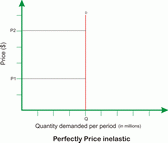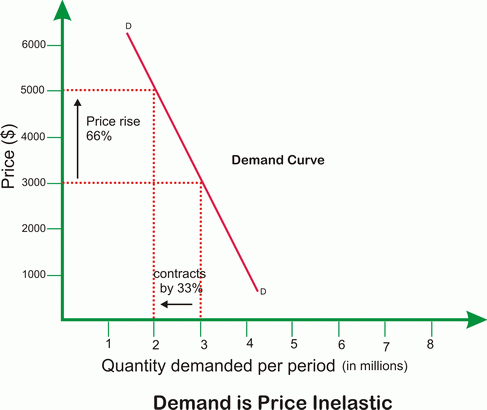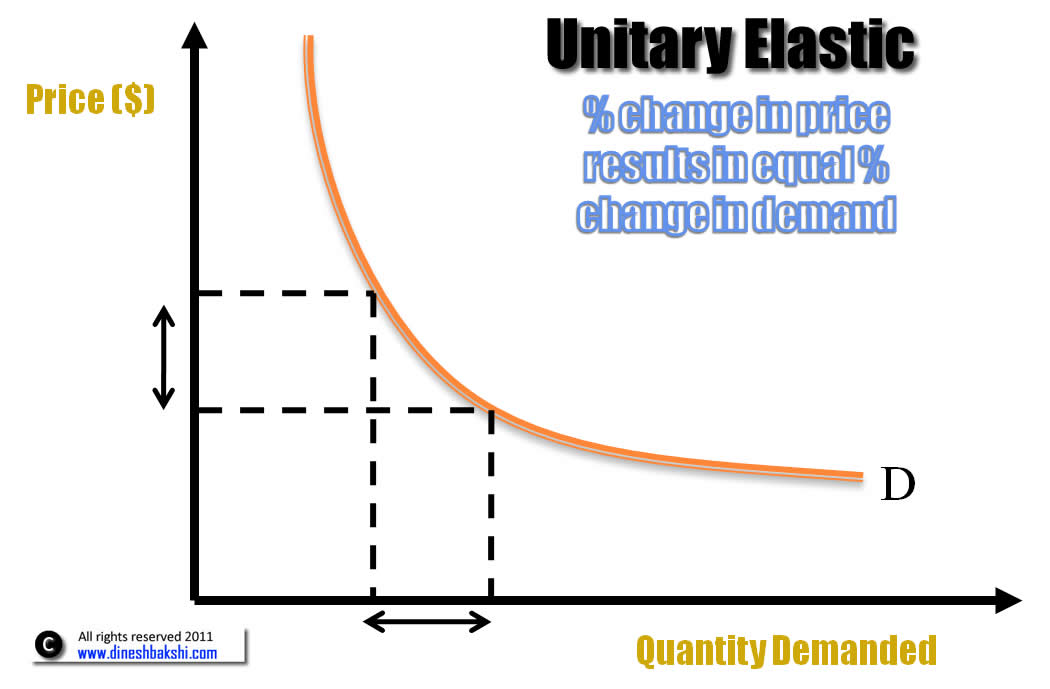# Price Elasticity of demand

The responsiveness of quantity demanded, or how much quantity demanded changes, given a change in the price of goods or service is known as the price elasticity of demand.

 Price Elasticity of demand (PED)= % change in quantity demanded % Change in price

## Negative sign

The mathematical value which is derived from the calculation is negative. A negative value indicates an inverse relationship between price and the quantity demanded. However, the negative sign is ignored.

## Range of PED

The value of PED might range from 0 to ?

Lets take a look at various types of PED.

### Perfectly Inelastic demandIn this case the PED =0 That means, any change in price will not have any effect on the demand of the product. Or in other words, the percentage change in demand will be equal to zero. It is hypothetical situation and does not exist in real world.

Perfectly elastic demand In this case the PED =?

The demand changes infinitely at a particular price. Any change in price will lead to fall of demand to zero. It is hypothetical situation and does not exist in real world.

However Normal goods have value of PED between 0 and ?. These can be classified as Inelastic demand When a product has a PED less than 1 and greater than 0, it is said to be have an inelastic demand. The percentage change in demand is less than the percentage change in price of the product.Demand for a product is said to be ELASTIC if the percentage change is demand is more than the percentage change in price.

The value of PED is more than 1.When there is a smaller percentage change in quantity demanded as compared to the percentage change in its price, the product is said to price INELASTIC.

The value of PED is less than 1.### Unitary Elastic Demand

When the percentage change in demand is equal to the percentage change in price, the product is said to have Unitary Elastic demand. In short, PED=1Watch a Video